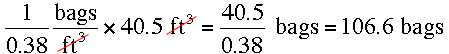SEARCH HOMEMath Central Quandaries & QueriesQuestion from Chris, a parent: I need 1 and one half yards of concrete for a project. If one bag is equal to .38 cubic feet, how many bags will I need?Hi Chris,

One cubic yard is 3 feet by 3 feet by 3 feet so its 3 × 3 × 3 = 27 cubic feet. Thus one and a half cubic yards is 1.5 × 27 = 40.5 cubic feet. At 0.38 cubic feet per bag that'sPennyMath Central is supported by the University of Regina and The Pacific Institute for the Mathematical Sciences.